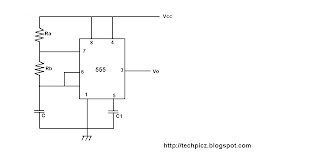## Tuesday, 21 August 2012

### ASTABLE MULTIVIBRATOR USING NE555

In this am going to explaining about astable multivibrator using ne555. Am already explained the astable multivibrator using BC107 and OPAMP in my previous post.The working of astable using ne555 is starting by the capacitor C.
Initially capacitor C starts charging through Ra and Rb towards Vcc with a time constant (Ra+Rb)C. Durring this time R=0, S=1, Q*=0 and ouput is high. When capacitor voltage equals (2/3)Vcc the upper comparator triggers the control flip flop so that Q*(Q bar)=1 . This makes transistor Q1 ON and the capacitor C starts discharging towards ground through Rb and transistor Q1 with a time constant RbC.

Durring thr discharge of the timing capacitor C, a it reaches Vcc/3 the lower comparator is triggerd and at this stage S=1, R=0, which turns Q*=0 . This makes transistor Q1 OFF and again capacitor C starts to charge. Thus the capacitor periodically charges and discharges between (2/3)Vcc . Output amplitude of the square wave swings between 0.3V to applied Vcc.

The charging periode of the capacitor Tc=0.69(Ra+Rb)C . The discharging periode of Td=0.69RbC . If Tc is equal to Td , the duty cycle of the output waveform will be 1/2 . In order to make Tc and Td equal to each other , a diode is connected in parallel with Rb such that capacitor charges through Ra and diode, and discharges through Rb . Another method is using a resistor of same value of Ra and Rb between the pin number 7 and the junction formed by Ra and Rb. The difference we can seen in the output waveform.Components for wiring this circuit

IC NE555
Ra - 6.8K
Rb - 6.8K
C - 0.1μF
C1 - 0.01μF
Vcc - 10V